# Cohort Estimator

## Definition

The Cohort Estimator is a simple frequentist estimation of multi-state transitions. The estimator can be used to derive the transition probability matrix of a Markov Chain process with a finite number of states.

## Methodology

• Prerequisite for applying the approach is the definition of a suitable number of temporal cohorts and the allocation of all observed entities into such cohorts. The temporal intervals defining the cohorts are part of the design of the statistical analysis and depend on the domain.
• A second methodological aspect is the treatment of left/right censoring and transitions happening within a cohort interval. Multiple approaches are possible (first or last observed state within a cohort, average or longest living state etc.)
• Whether the different periods / cohort intervals can be consider homogeneous or not.

## Single Period Cohort Estimator

In a single period cohort estimation all periods are considered equivalent (different samples of one-period transitions).

• The position in state space for an entity$i$ at discrete time$l$ is a random variable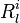$R^i_l$ taking values in the state space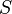$S$.
• We assume a finite state space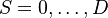$S ={0, \dots , D}$
• Cohorts are defined by equal temporal intervals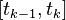$[t_{k-1}, t_{k}]$
• Let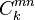$C^{mn}_k$ be the number (count) of entities at state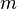$m$ at time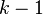$k-1$ and at state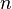$n$ at time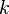$k$.
• Let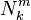$N^{m}_k$ be the number (count) of all entities at state$m$ at time$k-1$.

The directly estimated transition probability for that cohort is: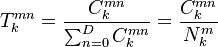$T^{mn}_{k} = \frac{C^{mn}_k}{ \sum_{n=0}^{D} C^{mn}_k} = \frac{C^{mn}_k}{N^{m}_{k}}$

The estimator reflects that the probability of transition from m to n is the observed count number$C^{mn}_k$ of all entities that migrated from m to n as a fraction of the count of all entities whose rating was m at k-1, that is,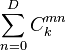$\sum_{n=0}^{D} C^{mn}_k$, irrespective of where they migrated to. The denominator includes all entities that did not migrate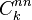$C^{nn}_k$.

### Count-Weighted Average

Under the assumption of homogeneity the estimates of different periods can be averaged. Using observation count as weights this results in an average transition matrix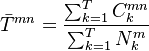$\bar{T}^{mn} = \frac{\sum_{k=1}^{T} C^{mn}_k}{\sum_{k=1}^{T} N^{m}_{k}}$

NB: The count-weighted average will in general be different that the simple arithmetic average of estimated matrices over different periods.

### Multi-Period Cohort Estimator

A multi-period estimator considers longer period intervals without the assumption of time-homogeneity. Computationally it is straight-forward extension: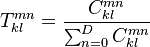$T^{mn}_{kl} = \frac{C^{mn}_{kl}}{\sum_{n=0}^{D} C^{mn}_{kl}}$

where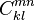$C^{mn}_{kl}$ denotes the migration count of the period [k,l].

## Confidence Intervals

Confidence intervals for the cohort estimator can be estimated using the multinomial proportions method

## Issues and Challenges

• State changes which occur within the period [k-1,k] are ignored. The time resolution (the number of cohorts) must be chosen so that there is as little ignored transition history as possible.
• The cohort estimator gives zero probability to a migration event that is not present in the data. E.g. in the presence of (right) censoring where we do not know what happens to the firm after the sample window closes (e.g. does it default right away or does it live on until the present) 
• Left truncation where firms only enter sample if they have either survived long enough or have received a rating.